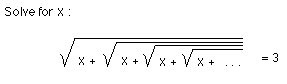## Sample Problems from Chapter 3

Chapter 3: Ian's Proof: Infinity = -1

1. Ian's problem- Ian came back one year from Winter break with this problem. He also brought me a copy of 'The Math Calendar' by Theoni Pappus, where he had seen the problem.2. Ian proves that infinity = -1
Using what you've figured out in chapter 1, can you write as a fraction the sum of this infinite series?
1 + a + a2 + a3 + a4 + a5 + ... = ?

To answers from Ch. 3
To order Don's materials
To choose sample problems from other chapters
Mathman home